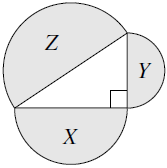## ksf-2015-st-09

Three semicircles have diameters which are the sides of a right-angled triangle.Their areas are $$X \text{ cm}^2$$, $$Y \text{ cm}^2$$ and $$Z \text{ cm}^2$$, as shown.

Question:

Which of the following is necessarily true?